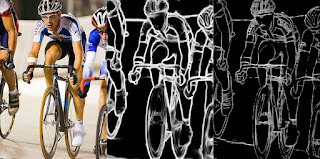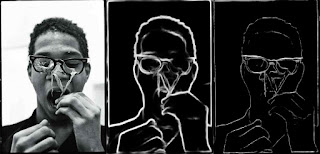## Thursday, 25 June 2020

### Dense extreme inception edge detection with opencv and deep learning

This tutorial introduce how to perform edge detection with opencv and deep learning. You will learn how to apply Dense Extreme Inception Network(DexiNed) and Holistically-Nested Edge Detection (HED) to images and videos. If you were interesting about HED, I recommend you study a brilliant post of pyimagesearch, I would explain the main points of how to perform edge detection by these networks with c++ and opencv.

## Apply HED by opencv and c++

This page explain how to do it,  you can find the link of the model and prototxt from there too. The author register a new layer, without it opencv cannot generate proper results.

## Apply DexiNed by opencv and c++

You can find out the explanation of DexiNed from this page, in order to perform edge detection by DexiNed, we need to convert the model to onnx, I prefer pytorch for this purpose. Why I do not prefer tensorflow? Because convert the model of tensorflow to the format opencv can read is much more complicated from my ex experiences, tensorflow is feature rich but I always feel like they are trying very hard to make things unnecessary complicated, their notoriously bad api design explain this very well.

### 1.Convert pytorch model of DexiNed to onnx

1. Clone the project blogCodes2
2. Navigate into edges_detection_with_deep_learning
3. Clone the project DexiNed
4. Copy the file model.py in edges_detection_with_deep_learning/model.py into DexiNed/DexiNed-Pytorch
5. Run the script to_onnx.py
If you do not want to go through the trouble, just download the model from here.

### 2.Load and forward image by DexiNed and HED

```void switch_to_cuda(cv::dnn::Net &net)
{
try {
for(auto const &vpair : cv::dnn::getAvailableBackends()){
std::cout<<vpair.first<<", "<<vpair.second<<std::endl;
if(vpair.first == cv::dnn::DNN_BACKEND_CUDA && vpair.second == cv::dnn::DNN_TARGET_CUDA){
std::cout<<"can switch to cuda"<<std::endl;
net.setPreferableBackend(cv::dnn::DNN_BACKEND_CUDA);
net.setPreferableTarget(cv::dnn::DNN_TARGET_CUDA);
break;
}
}
}catch(std::exception const &ex){
net.setPreferableBackend(cv::dnn::DNN_BACKEND_DEFAULT);
net.setPreferableTarget(cv::dnn::DNN_TARGET_CPU);
throw std::runtime_error(ex.what());
}
}

std::tuple<cv::Mat, long long> forward_utils(cv::dnn::Net &net, cv::Mat const &input, cv::Size const &blob_size)
{
using namespace std::chrono;

//measure duration
auto const start = high_resolution_clock::now();
cv::Mat blob = cv::dnn::blobFromImage(input, 1.0, blob_size,
cv::Scalar(104.00698793, 116.66876762, 122.67891434), false, false);
net.setInput(blob);
cv::Mat out = net.forward();
cv::resize(out.reshape(1, blob_size.height), out, input.size());
//the data type of out is CV_32F(single channel, floating point) so we need to upscale the value and convert
//it to CV_8U(single channel, uchar)
out *= 255;
out.convertTo(out, CV_8U);
//convert gray to bgr because we need to create montage(1 row, 3 column of images in our case)
auto const finish = high_resolution_clock::now();
auto const elapsed = duration_cast<milliseconds>(finish - start).count();
cv::cvtColor(out, out, cv::COLOR_GRAY2BGR);

return {out, elapsed};
}

class hed_edges_detector
{
public:
hed_edges_detector(std::string const &weights, std::string const &config) :
{
switch_to_cuda(net_);
}

long long elapsed() const
{
return elapsed_;
}

cv::Mat forward(cv::Mat const &input)
{
auto result = forward_utils(net_, input, {500, 500});
elapsed_ += std::get<1>(result);
return std::get<0>(result);
}

private:
long long elapsed_ = 0;
cv::dnn::Net net_;
};

class dexi_edges_detector
{
public:
explicit dexi_edges_detector(std::string const &model) :
{
switch_to_cuda(net_);
}

long long elapsed() const
{
return elapsed_;
}

cv::Mat forward(cv::Mat const &input)
{
auto result = forward_utils(net_, input, {400, 400});
elapsed_ += std::get<1>(result);
return std::get<0>(result);
}

private:
long long elapsed_ = 0;
cv::dnn::Net net_;
};
```

### 3. Detect edges of image

```void test_image(std::string const &mpath)
{
hed_edges_detector hed(mpath + "hed_pretrained_bsds.caffemodel", mpath + "deploy.prototxt");
auto hed_out = hed.forward(img);

dexi_edges_detector dexi(mpath + "24_model.onnx");
auto dexi_out = dexi.forward(img);

cv::Size const frame_size(img.cols, img.rows);
int constexpr grid_x = 3;
int constexpr grid_y = 1;
ocv::montage mt(frame_size, grid_x, grid_y);

cv::imshow("results", mt.get_montage());
cv::imwrite("results2.jpg", mt.get_montage());
cv::waitKey();
}
```

### 4. Detect edges of video

```void test_video(std::string const &mpath)
{
cv::VideoCapture cap("pedestrian.mp4");
if(cap.isOpened()){
hed_edges_detector hed(mpath + "hed_pretrained_bsds.caffemodel", mpath + "deploy.prototxt");
dexi_edges_detector dexi(mpath + "24_model.onnx");

//unique_ptr is a resource manager class(smart pointer) of c++,
//we allocate memory by the reset(or make_unique) api,
//after leaving the scope(scope is surrounded by {}), the memory will be released. In c++, the
//best way of manage the resource is avoid explicit memory allocation, if you really need to do it,
//guard your memory by smart pointer. I use unique_ptr at here because I cannot
//initialize the objects before I know the frame size of the video.
std::unique_ptr<ocv::montage> mt;
std::unique_ptr<cv::VideoWriter> vwriter;

cv::Mat frame;
float frame_count = 0;
while(1){
cap>>frame;
if(frame.empty()){
break;
}

++frame_count;
cv::resize(frame, frame, {}, 0.5, 0.5);
auto const hed_out = hed.forward(frame);
auto const dexi_out = dexi.forward(frame);
if(!mt){
//initialize the class to create montage
//First arguments tell the class the size of each frame
cv::Size const frame_size(frame.cols, frame.rows);
int constexpr grid_x = 3;
int constexpr grid_y = 1;
mt.reset(new ocv::montage(frame_size, grid_x, grid_y));
}
if(!vwriter){
auto const fourcc = cv::VideoWriter::fourcc('F', 'M', 'P', '4');
int constexpr fps = 30;
//because the montage is 3 columns and 1 row, so the cols need to multiply by 3
vwriter.reset(new cv::VideoWriter("out.avi", fourcc, fps, {frame.cols * 3, frame.rows}));
}

auto const montage = mt->get_montage();
cv::imshow("out", mt->get_montage());
vwriter->write(montage);
cv::waitKey(10);
mt->clear();
}
std::cout<<"hed elapsed time = "<<hed.elapsed()<<", frame count = "<<frame_count
<<", fps = "<<1000.0f/(hed.elapsed()/frame_count)<<std::endl;
std::cout<<"dexi elapsed time = "<<dexi.elapsed()<<", frame count = "<<frame_count
<<", fps = "<<1000.0f/(dexi.elapsed()/frame_count)<<std::endl;
}else{
std::cerr<<"cannot open video pedestrian.mp4"<<std::endl;
}
}
```

## Results of image detectionimg_00img_01

## Runtime performance on gpu(gtx 1060)

Following results are based on the video I posted on youtube.The video has 733 images. From left to right is original frame, frame processed by HED, frame processed by DexiNed.

HED elapsed time is 43870ms, fps is 16.7085.
DexiNed elapsed time is 45149ms, fps is 16.2351.

The crop layer of HED do not support cuda, it should become faster after the cuda layer is done.

## Source codes

Located at github.